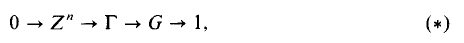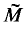Home

# Decomposition of flat manifolds

## Extract

Let M be a compact flat Riemannian manifold of dimension n, and Γ its fundamental group. Then we have the following exact sequence (see )where Zn is a maximal abelian subgroup of Γ and G is a finite group isomorphic to the holonomy group of M. We shall call Γ a Bieberbach group. Let T be a flat torus, and let Ggr act via isometries on T; then ┌ acts isometrically on× T whereis the universal covering of M and yields a flat Riemannian structure on (× T)/Γ. A flat-toral extension (see [9, p. 371]) of the Riemannian manifold M is any Riemannian manifold isometric to (× T)/Γ where T is a flat torus on which Γ acts via isometries. It is convenient to adopt the convention that a single point is a 0-dimensional flat torus. If this is done, M is itself among the flat toral extensions of M. Roughly speaking, this is a way of putting together a compact flat manifold and a flat torus to make a new flat manifold the dimension of which is the sum of the dimensions of its constituents. It is, more precisely, a fibre bundle over the flat manifold with a flat torus as fibre.

## References

Hide All
MathJax
MathJax is a JavaScript display engine for mathematics. For more information see http://www.mathjax.org.

# Decomposition of flat manifolds

## Metrics

### Full text viewsFull text views reflects the number of PDF downloads, PDFs sent to Google Drive, Dropbox and Kindle and HTML full text views.

Total number of HTML views: 0
Total number of PDF views: 0 *Loading metrics...

### Abstract viewsAbstract views reflect the number of visits to the article landing page.

Total abstract views: 0 *Loading metrics...

* Views captured on Cambridge Core between <date>. This data will be updated every 24 hours.

Usage data cannot currently be displayed